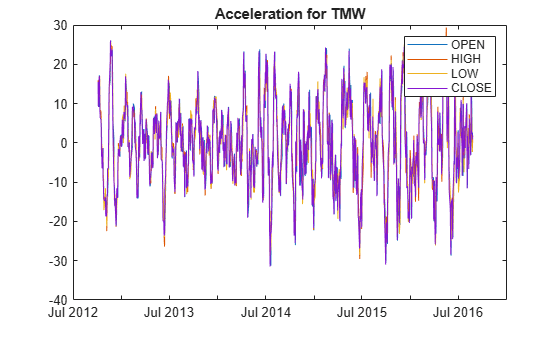# tsaccel

Acceleration between times

Using a `fints` object for the `Data` argument of `tsaccel` is not recommended. Use a vector, matrix, `timetable`, or `table` instead for financial time series. For more information, see Convert Financial Time Series Objects fints to Timetables.

## Syntax

``acceleration = tsaccel(Data)``
``acceleration = tsaccel(___,Name,Value)``

## Description

example

````acceleration = tsaccel(Data)` calculates the acceleration of a data series with time distance of n periods.n periods.```

example

````acceleration = tsaccel(___,Name,Value)` adds optional name-value pair arguments. ```

## Examples

collapse all

Load the file `SimulatedStock.mat`, which provides a timetable (`TMW`) for financial data for TMW stock.

```load SimulatedStock.mat TMW.Volume = []; % remove VOLUME field acceleration = tsaccel(TMW); plot(acceleration.Time,acceleration.Variables) legend('OPEN','HIGH','LOW','CLOSE') title('Acceleration for TMW')```## Input Arguments

collapse all

Data with high, low, open, close information, specified as a vector, matrix, table, or timetable. For vector input, `Data` is a column vector of high, low, open, and closing prices stored in the corresponding columns. For matrix input, `Data` is an `M`-by-`N` column-oriented matrix of high, low, open, and closing prices stored in the corresponding columns. Timetables and tables with `M` rows must contain variables named `'High'`, `'Low'`, `'Open'`, and `'Close'` (case insensitive).

Data Types: `double` | `table` | `timetable`

### Name-Value Arguments

Specify optional pairs of arguments as `Name1=Value1,...,NameN=ValueN`, where `Name` is the argument name and `Value` is the corresponding value. Name-value arguments must appear after other arguments, but the order of the pairs does not matter.

Before R2021a, use commas to separate each name and value, and enclose `Name` in quotes.

Example: ```accelearation = tsaccel(TMW,'NumPeriods',10,'Datatype',1)```

Period difference for acceleration, specified as the comma-separated pair consisting of `'NumPeriods'` and a scalar positive integer.

Data Types: `double`

Indicates if `Data` contains the data itself or the momentum of the data, specified as the comma-separated pair consisting of `'Datatype'` and a scalar integer with a value of:

• `0``Data` contains the data itself.

• `1``Data` contains the momentum of the data.

Data Types: `double`

## Output Arguments

collapse all

Acceleration series, returned with the same number of rows (`M`) and columns (`N`) and the same type (vector, matrix, table, or timetable) as the input `Data`.